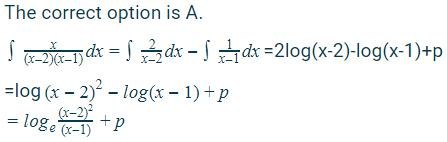# Test: Integration By Partial Fractions

## 10 Questions MCQ Test Mathematics (Maths) Class 12 | Test: Integration By Partial Fractions

Description
This mock test of Test: Integration By Partial Fractions for JEE helps you for every JEE entrance exam. This contains 10 Multiple Choice Questions for JEE Test: Integration By Partial Fractions (mcq) to study with solutions a complete question bank. The solved questions answers in this Test: Integration By Partial Fractions quiz give you a good mix of easy questions and tough questions. JEE students definitely take this Test: Integration By Partial Fractions exercise for a better result in the exam. You can find other Test: Integration By Partial Fractions extra questions, long questions & short questions for JEE on EduRev as well by searching above.
QUESTION: 1

Solution:
QUESTION: 2

Solution:
QUESTION: 3

### Evaluate: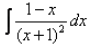Solution:
QUESTION: 4

Evaluate: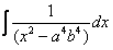Solution:

∫dx/(x2 - a4b4)
= ∫dx/(x2 - a2b2)2
= ∫dx/(x2 - a2b2)(x2 + a2b2)
As by formula dx/(n - n)(n + n) = 1/2n log|(x - n)/(x + n)|
= 1/2n log|(x - a2b2)/(x + a2b2)|

QUESTION: 5

Simplify the integrand of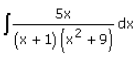Solution:

Let I = ∫5x dx/[(x+1)(x2+9)]
Let 5x/[(x+1)(x2+9)] = A(x+1) + (Bx + C)/(x2+9)
⇒5x = (A+B)x2 + (B+C)x + 9A + C
Comparing the coefficients of x2 on both sides, we get
A + B = 0    ...(1)
Comparing the coefficients of x on both sides, we get
B + C = 5    .....(2)
Comparing constants on both sides, we get
9A +C = 0    ....(3)
From (1), we get B = −A
From (3), we get C = −9A
now, from (2), we get
−A − 9A = 5
⇒A = −1/2
B = 1/2
C = 9/2
So, 5x/[(x+1)(x2+9)] = −1/2 × [1(x+1)] + 1/2 × (x + 9)/(x+ 9)
So, ∫5x dx/[(x+1)(x+ 9)] = −1/2∫dx/(x+1) + 1/2∫(x+9)/(x+9) dx
=−1/2 log|x+1| + 1/2∫x dx/(x2 +9) + 9/2∫dx (x+9)
=−1/2 log|x+1| + 1/4∫2x dx/(x+9) + 9/2∫dx/(x+(3)2)
⇒−1/2 log |x+1| + 1/4log|x 2 +9| + 9/2×1/3 tan−1(x/3) + C
=−1/2 log |x+1| + 1/4log∣|x2+9| + 3/2 tan−1(x/3) + C

QUESTION: 6

Evaluate: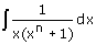Solution:

∫dx/x(xn + 1)..............(1)
∫dx/x(xn + 1) *(xn - 1)/(xn - 1)
Put xn = t
dt = nx(n-1)dx
dt/n = x(n-1)dx
Put the value of dt/n in eq(1)
= ∫(1/n)dt/t(t+1)
= 1/n ∫dt/(t+1)t
= 1/n{∫dt/t -  ∫dt/t+1}
= 1/n {ln t - lnt + 1} + c
= 1/n {ln |t/(t + 1)|} + c
= 1/n {ln |xn/(xn + 1)|} + c

QUESTION: 7

How would you split the rational function f(x) into partial function?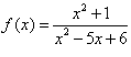Solution:
QUESTION: 8

The integral of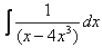is:

Solution:

∫dx/x3(x-2 -4).............(1)
=  ∫x-3 dx/(x-2 - 4)​
Let t = (x-2 - 4)
dt = -2x-3 dx
x-3 = -dt/2
Put the value of x-3 in eq(1)
= -½  ∫dt/t
= -½ log t + c
= -½ log(x-2 - 4) + c
= -½ log(1-4x2)/x2 + c
= ½ log(x2/(1 - 4x2)) + c

QUESTION: 9

The value of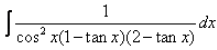Solution:

∫1/cos2 x(1 - tanx)(2 - tanx) dx
= ∫(sec2 x)/(2 - 3tanx + tan2x) dx
Put t = tanx
dt = sec2x dx
∫dt/(2 - 3t + t2)dx
∫dt/(t2 - 3t + 9/4) + (2 - 9/4)
= ∫dt/((t - 3/2)2 - (½)
Using the formula ∫dx/(x2 - a2), we get
= ½[½] log |[t - 3/2 - ½]/[t - 3/2 + ½]| + c
= log|(t - 2)/(t - 1)| + c
Taking - common, we get
= log|(2 - t)/(1 - t)| + c
log|(2 - tanx)/(1 - tanx)| + c

QUESTION: 10

Correct evaluation of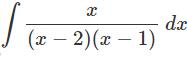Solution: